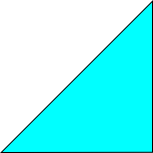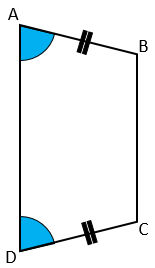# Exam-Style Questions on Proof

## Problems on Proof adapted from questions set in previous Mathematics exams.

### 1.

GCSE Higher

State whether each of the following statements is true or false. Give reasons for your answers.

(a) When $$a^2 = 25$$ the only value that $$a$$ can have is 5.

(b) When $$b$$ is a positive integer, the value of $$3b$$ is always a factor of the value of $$12b$$.

(c) When $$c$$ is positive, the value of $$c^2$$ is always greater than the value of $$c$$.

### 2.

GCSE Higher

One is added to the product of two consecutive positive even numbers. Show that the result is a square number.

### 3.

GCSE Higher

(a) Give a reason why 0 is an even number.

(b) The lengths of the sides of a right-angled triangle are all integers. Prove that if the lengths of the two shortest sides are even, then the length of the third side must also be even.### 4.

GCSE Higher

Betsy thinks that $$(3x)^2$$ is always greater than or equal to $$3x$$.

Is she is correct?

### 5.

GCSE Higher

Given that $$n$$ can be any integer such that $$n \gt 1$$, prove that $$n^2 + 3n$$ is even.

### 6.

GCSE Higher

Use algebra to prove that $$0.3\dot1\dot8 \times 0.\dot8$$ is equal to $$\frac{28}{99}$$.

### 7.

GCSE Higher

The diagram shows a quadrilateral ABCD in which angle DAB equals angle CDA and AB = CD.Prove that the diagonals of this quadrilateral are of equal length.

### 8.

GCSE Higher

m and n are positive whole numbers with m > n

m2 – n2 = (m + n)(m – n)

If m2 – n2 is a prime number prove that m and n are consecutive numbers.

### 9.

GCSE Higher

(a) Prove that the recurring decimal $$0.\dot2 \dot1$$ has the value $$\frac{7}{33}$$

(b) The value of $$x$$ is given as:

$$x=\frac{1}{5^{120}\times2^{123}}$$

Show that, when $$x$$ is written as a terminating decimal, there are 120 zeros after the decimal point.

(c) The reciprocal of any prime number $$p$$ (where $$p$$ is neither 2 nor 5) when written as a decimal, is always a recurring decimal.

A theorem in mathematics states:

The period of a recurring decimal is the least value of $$n$$ for which $$p$$ is a factor of $$10^n – 1$$

Marilou tests this theorem for the reciprocal of eleven.

She uses her calculator to show that 11 is a factor of $$10^2 – 1$$ then makes this statement:

"The period of the recurring decimal is 2 because 11 is a factor of $$10^2-1$$. This shows the theorem to be true in this case."

Explain why Marilou's statement is incomplete.

### 10.

GCSE Higher

$$\frac{p-1}{q-1}-\frac pq$$

Using your answer to part (a), prove that if $$p$$ and $$q$$ are positive integers and $$p \lt q$$, then

$$\frac{p-1}{q-1}-\frac pq\lt 0$$

### 11.

GCSE Higher

(a) Prove that the product of two consecutive whole numbers is always even.

(b) Prove, by giving a counter example, that the sum of four consecutive integers is not always divisible by 4.

### 12.

GCSE Higher

Prove that the expression below is always positive.

$$x^2 - 5x + 9$$

### 13.

IB Analysis and Approaches

Consider two consecutive positive even numbers, $$2n$$ and $$2n + 2$$.

Show that the difference of their squares is equal to twice their sum.

### 14.

IB Analysis and Approaches

(a) Show that $$(2n + 1)^2 + (2n + 3)^2 = 8n^2 +16n + 10$$ , where $$n \in \mathbb{Z}$$

(b) Hence, or otherwise, prove that the sum of the squares of any two consecutive odd integers is even.

If you would like space on the right of the question to write out the solution try this Thinning Feature. It will collapse the text into the left half of your screen but large diagrams will remain unchanged.

The exam-style questions appearing on this site are based on those set in previous examinations (or sample assessment papers for future examinations) by the major examination boards. The wording, diagrams and figures used in these questions have been changed from the originals so that students can have fresh, relevant problem solving practice even if they have previously worked through the related exam paper.

The solutions to the questions on this website are only available to those who have a Transum Subscription.

Exam-Style Questions Main Page

Search for exam-style questions containing a particular word or phrase:

To search the entire Transum website use the search box in the grey area below.